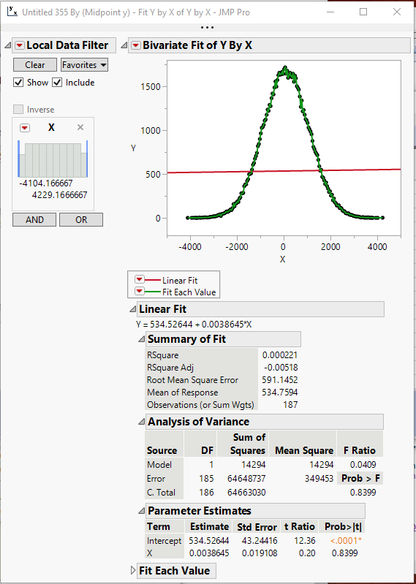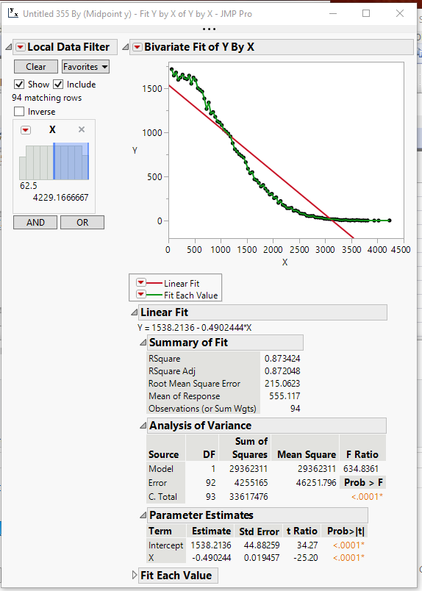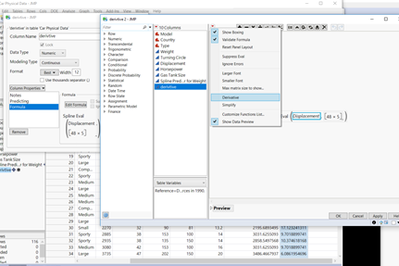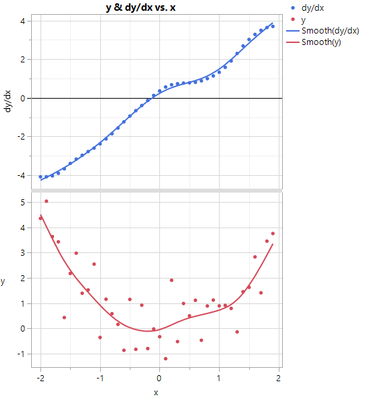Our World Statistics Day conversations have been a great reminder of how much statistics can inform our lives. Do you have an example of how statistics has made a difference in your life? Share your story with the Community!
Choose Language Hide Translation Bar
Highlighted

## Slopes

Hello everyone,

I have a graph of this sort (attached Image) and I want to find M (slope) of the drop from the peak. How can I possibly find the slopes of these two on JMP?

Pls help, Thank you

1 ACCEPTED SOLUTION

Accepted Solutions
Highlighted

## Re: Slopes

The easiest way to do this, is to use the Fit Y by X platform, and have it draw your curve and then to select

1. Fit Each Value
2. Fit Line
3. Select Local Data Filter and select the X value to be filtered

Here are the results with my example dataNote that under the Parameter Estimates you can see the slope of .0038645

Now all you have to do, is to use the Data Filter to select only the values on the left side of the graph.The regression now is only calculated on the down slope, which is -0.490244

Jim
8 REPLIES 8
Highlighted

## Re: Slopes

You should be able to accomplish this, by excluding the data points on the left side of the curve, and then regressing the values from the peak value to the lowest value on the right.

For the poly curve line, you could use this technique multiple times.

Jim
Highlighted

## Re: Slopes

So sorry, Please this is not so clear to me. How do I regress the value from the peak to the lowest?

Highlighted

## Re: Slopes

The easiest way to do this, is to use the Fit Y by X platform, and have it draw your curve and then to select

1. Fit Each Value
2. Fit Line
3. Select Local Data Filter and select the X value to be filtered

Here are the results with my example dataNote that under the Parameter Estimates you can see the slope of .0038645

Now all you have to do, is to use the Data Filter to select only the values on the left side of the graph.The regression now is only calculated on the down slope, which is -0.490244

Jim
Highlighted

## Re: Slopes

If you know the function of the curves or just do bivariate fit -spline fit and then save the prediction formula. Then do the derivative of the curve function under formula editor, screen shot below. you will have slopes at any Xs.Highlighted

## Re: Slopes

I tried this but it is giving multiple values for each date but I want just one that explains the drop...

Highlighted

## Re: Slopes

you can make a plot of dy/dx vs x. you can find where dy/dx=0, that's where y starts from decrease to increase in my example below.Highlighted

## Re: Slopes

You need to explain this a bit more....I don't understand what you are saying. Can you show what you mean about the issue with multiple date values. If what you mean is that you have two or more groups in your data as shown in your attached pgn file, then all you have to do, is to specify to group the data and then JMP will provide separate slopes for each group.
Jim
Highlighted

## Re: Slopes

Yes, I have two groups, so what I am doing now is to separate them into two bivariate graphs instead of the way it looks in the initial image I sent. I won't my two know how it can be both represented using Bivariate and not graph builder. Thanks for all the response, it is really helping
Article Labels